猫猫头钢琴——CatPiano背景介绍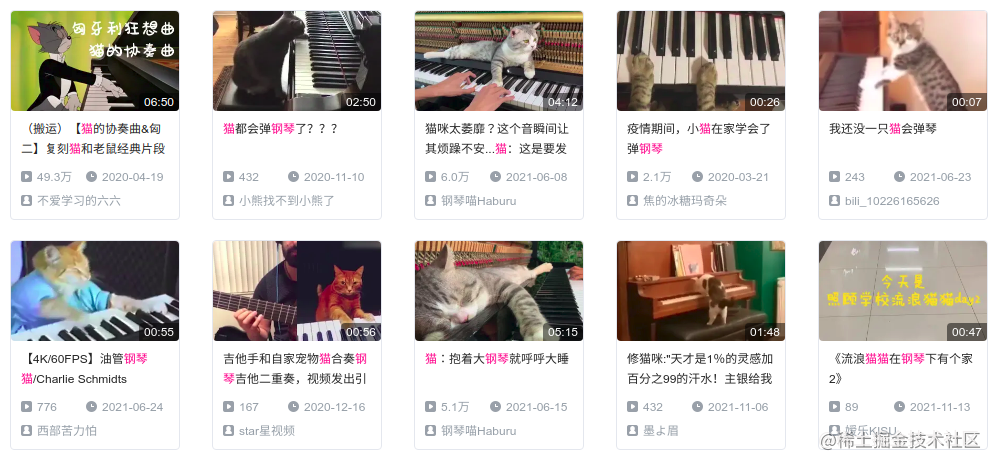（猫主子：就是这么糊弄劳资的吗）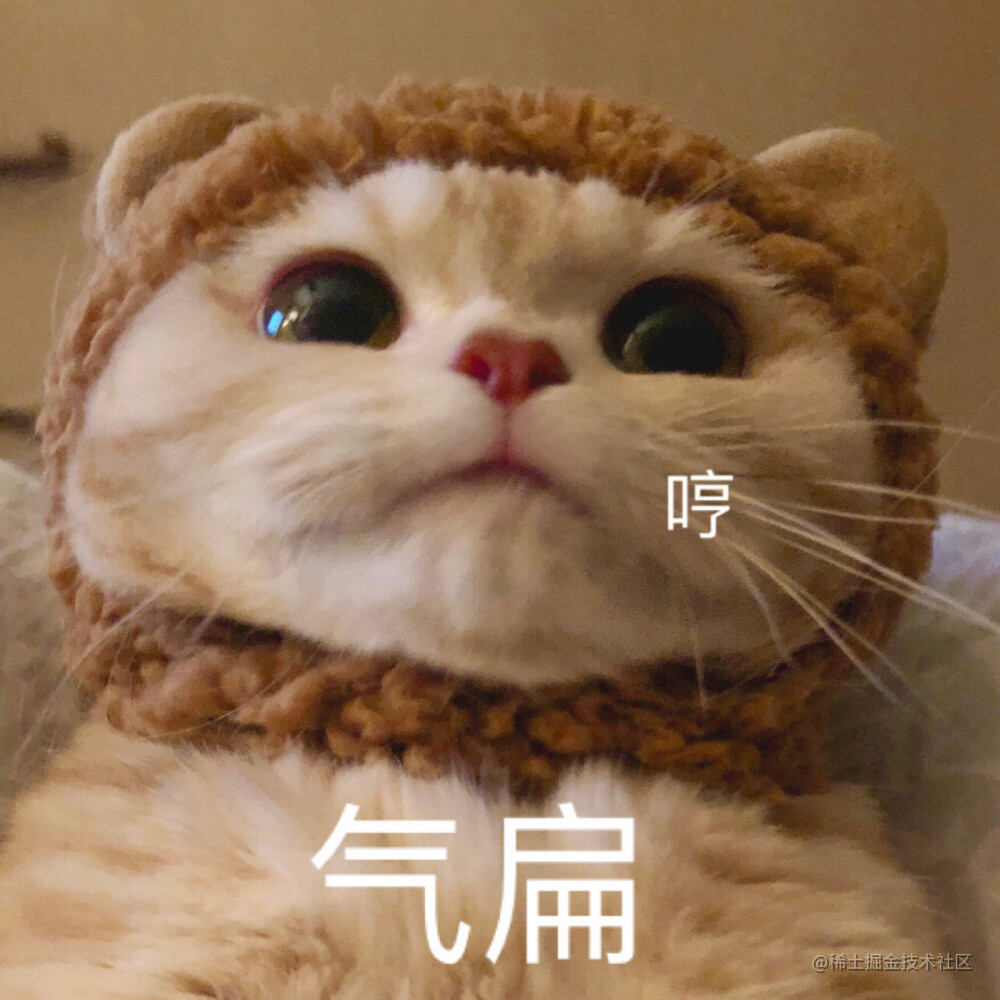• 怎么拍摄猫主子的视频
• 怎么检测猫头的位置
• 怎么弹琴与怎么显示

图像采集与标注

（猫主子：爷这么可爱不给爷喝一口的吗）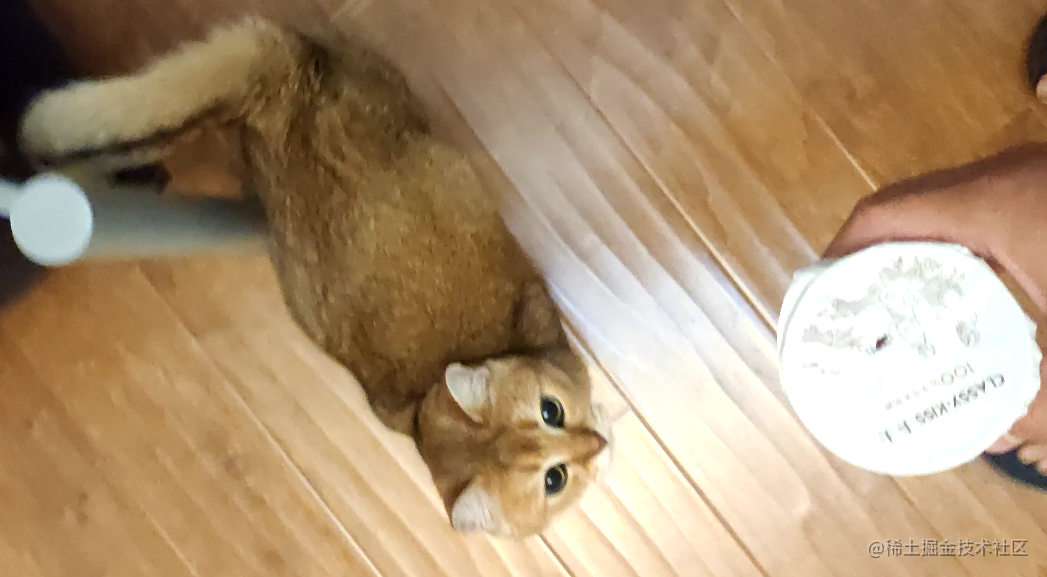• 众所周知，猫是流体，因此标注猫身子并不利于检测，因此最终选择标注猫头
• 因为背景简单且任务单一，所以标注少量图片即可完成猫头检测
• 标注时需要注意标注的格式，务必要采用 YOLOV5 模型可以识别的模式进行标注

YOLOV5 模型训练

模型训练

python .\train.py --data .\data\cat.yaml --weights .\weights\yolov5s.pt --img 160 --epochs 3000

模型测试

模型前期训练效果图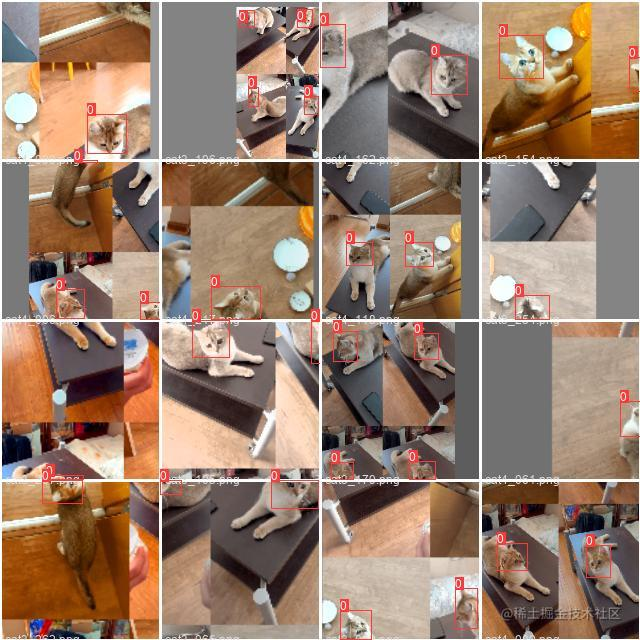模型前期验证效果图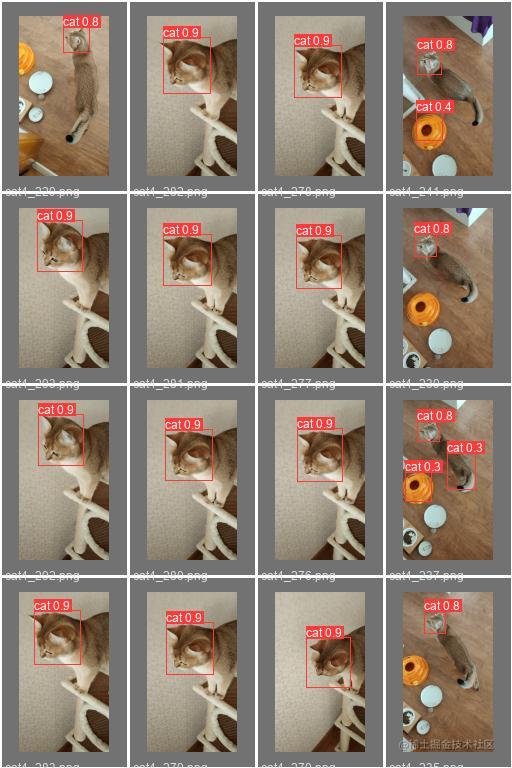推理部署

推理框架安装

git clone https://github.com/openppl-public/ppl.nn.git
cd ppl.nn
./build.sh -DHPCC_USE_X86_64=ON -DHPCC_USE_OPENMP=ON -DPPLNN_ENABLE_PYTHON_API=ON

模型推理

def RegisterEngines():
engines = []

# create x86 engine
x86_options = pplnn.X86EngineOptions()
x86_engine = pplnn.X86EngineFactory.Create(x86_options)

engines.append(pplnn.Engine(x86_engine))
return engines

class ModelRunner(object):
def __init__(self, model_path):
self.__initialize(model_path)

def __initialize(self, model_path):
# register engines
engines = RegisterEngines()
if len(engines) == 0:
raise Exception('failed to register engines')

# create runtime builder
runtime_builder = pplnn.OnnxRuntimeBuilderFactory.CreateFromFile(model_path, engines)
if not runtime_builder:
raise Exception('failed to create runtime builder from file: %s' % (model_path))

# create runtime
self.runtime = runtime_builder.CreateRuntime()
if not self.runtime:
raise Exception('failed to create runtime')

def get_input_tensor_shape(self):
return self.runtime.GetInputTensor(0).GetShape().GetDims()

def forward(self, input):
if not self.runtime:
raise Exception('runtime not created')

# get input tensor info
tensor = self.runtime.GetInputTensor(0)
shape = tensor.GetShape()
np_data_type = g_pplnntype2numpytype[shape.GetDataType()]
dims = shape.GetDims()

# feed input data
input = np.ascontiguousarray(input) # use contiguousarray to avoid calc error
status = tensor.ConvertFromHost(input)
if status != pplcommon.RC_SUCCESS:
raise Exception('failed to set input data')

# start to inference
status = self.runtime.Run()
if status != pplcommon.RC_SUCCESS:
raise Exception('failed to run')

# wait for inference finished
status = self.runtime.Sync()
if status != pplcommon.RC_SUCCESS:
raise Exception('failed to sync')

# get output data
out_datas = {}
for i in range(self.runtime.GetOutputCount()):
# get output tensor info
tensor = self.runtime.GetOutputTensor(i)
tensor_name = tensor.GetName()
# fetch output data
tensor_data = tensor.ConvertToHost()
if not tensor_data:
raise Exception('failed to get output ' + tensor_name)

out_data = np.array(tensor_data, copy=False)
out_datas[tensor_name] = copy.deepcopy(out_data)

return out_datas

数据预处理与后处理

# preprocess
img = cv2.resize(img, (self.input_img_w, self.input_img_h)) # resize
img = cv2.cvtColor(img, cv2.COLOR_BGR2RGB)                  # BGR -> RGB
img = img.transpose(2, 0, 1)                                # HWC -> CHW
img = img.astype(dtype = np.float32)                        # uint8 -> fp32
img /= 255                                                  # normalize
img = np.expand_dims(img, axis=0)                           # add batch dimension

制作琴键

琴键声音

pip3 install mingus
pip3 install fluidsynth

from mingus.midi import fluidsynth
fluidsynth.init('/usr/share/sounds/sf2/FluidR3_GM.sf2', 'alsa')    # for ubuntu
fluidsynth.play_Note(64, 0, 100)                                   # 标准音 a1

键盘显示

class KeyType(Enum):
WHITE_KEY = 0,
WHITE_KEY_LEFT = 1,
WHITE_KEY_RIGHT = 2,
BLACK_KEY = 3

PianoKey 中有一个 play(self, position) 接口，一旦 position 落在了琴键的范围内，就认定琴键被按下，发出琴键对应的声音。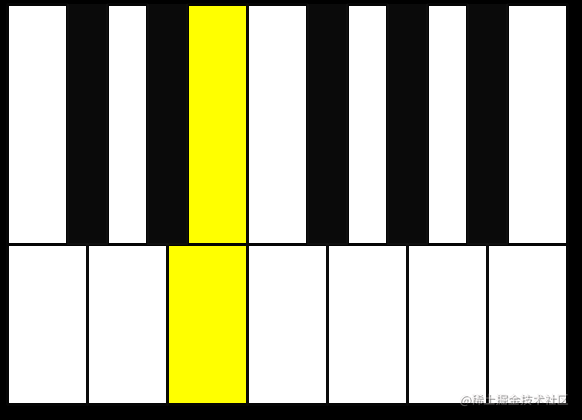最终效果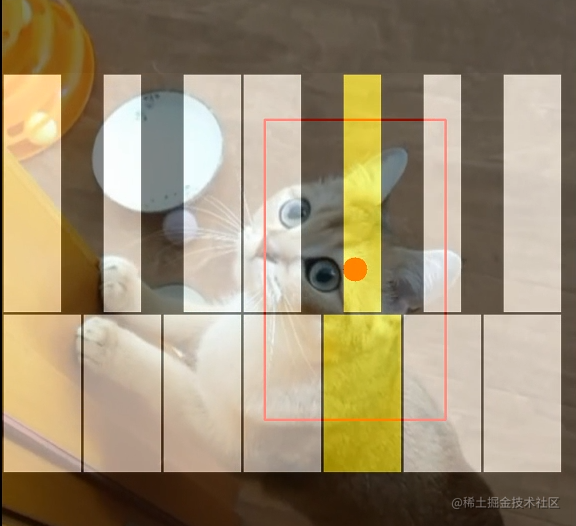视频 demo

github repo 链接：github.com/ZichenTian/…